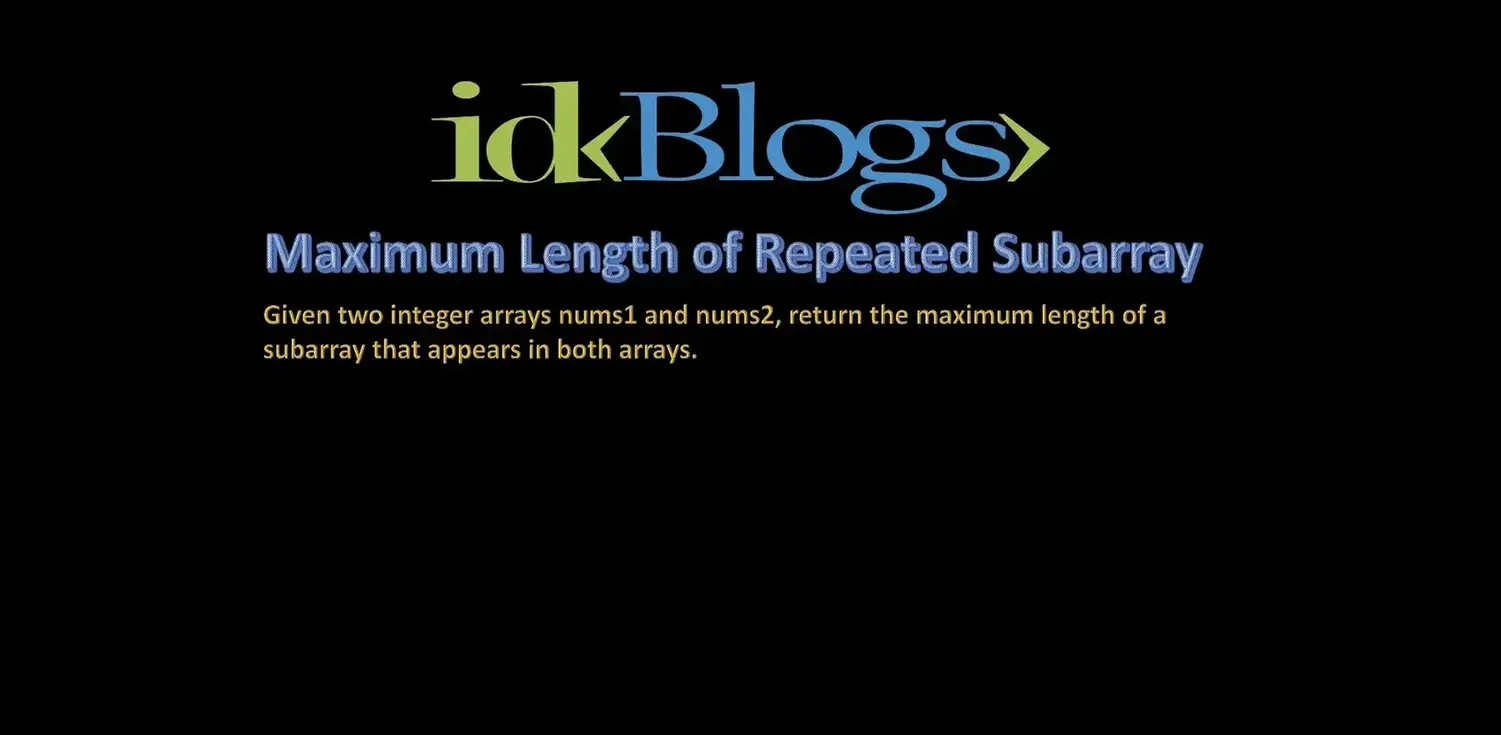# Maximum Length of Repeated Subarray

## Given two integer arrays nums1 and nums2, return the maximum length of a subarray that appears in both arrays.

Shubham Verma      July 7, 2021## Get the maximum length of repeated subarray

### Example 1:

Input: nums1 = [1,2,3,2,1], nums2 = [3,2,1,4,7]
Output: 3
Explanation: The repeated subarray with maximum length is [3,2,1].

### Example 2:

Input: nums1 = [0,0,0,0,0], nums2 = [0,0,0,0,0]
Output: 5

### Constraints:

1 <= nums1.length, nums2.length <=1000
0 <= nums1[i], nums2[i] <=100

# Solution:

``````                                    var findLength = function(A,B) {

let n=A.length;
let m=B.length

let dp = new Array(n + 1);
for (let i = 0; i <= n; i++)
{
dp[i]=new Array(m+1);
for (let j = 0; j <= m; j++)
dp[i][j] = 0;
}

for (let i = n - 1; i >= 0; i--)
{
for (let j = m - 1; j >= 0; j--)
{
if (A[i] == B[j]){
let xxx=dp[j + 1][i + 1];
if(!xxx){
xxx=0;
}
dp[j][i] = xxx + 1;
}
}
}
let maxm = 0;
for (let i = 0; i < n; i++)
{
for (let j = 0; j < m; j++)
{
maxm = Math.max(maxm, dp[i][j]);
}
}

return maxm;
};

``````

### Output:Given two integer arrays nums1 and nums2, return the maximum length of a subarray that appears in both arrays.

# For Other Countries (United States, United Kingdom, Spain, Italy, Germany, France, Canada, etc)

Thank you

To read more about the technologies, Please subscribe us, You'll get the monthly newsletter having all the published article of the last month.# 👈

## Enjoyed this moment?

### OTHER POSTS